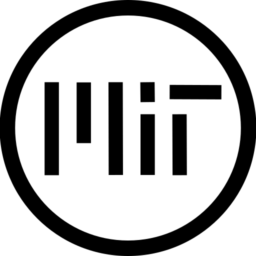# 3D Vertical dissolve

To make it work properly you need to set the global_transform shader parameter via GDScript. This is needed as the value cannot be calculated via the shader itself.

The shader is fully customisable and can be used to dissolve the mesh or blend another texture to have for example snow/moss effects on rocks.

• offset – is the dissolve offset
• up – if true the offset is applied from the top part of the mesh, otherwise from the bottom one
• borderColor – the border colour of the dissolve
• waveAmplitude   the amplitude of the sin waves
• wavePhase – the phase of the sin waves
• emissionIntensity – set the intensity of the border color
• noiseSpeed – the speed of the noise effect
• noiseInfluence – how much noise to apply, 0 for none
• textureUVScale – the texture to blend, use a transparent one if you want just a dissolve effect
``````shader_type spatial;
render_mode cull_disabled;

uniform sampler2D texture;
uniform sampler2D blendTexture;
uniform sampler2D noiseTexture;

uniform float offset = 0.;
uniform bool up = true;
uniform vec4 borderColor: hint_color = vec4(1., 1., 0., 1.);
uniform float borderHeight = 0.1;
uniform float waveAmplitude = 1.;
uniform float waveFrequency = 1.;
uniform float wavePhase = 0.1;
uniform float emissionIntensity = 1.;
uniform float noiseSpeed = .01;
uniform float noiseInfluence = 1.;

uniform vec2 blendUVScale = vec2(1.);
uniform vec2 noiseUVScale = vec2(1.);
uniform vec2 textureUVScale = vec2(1.);

const float tao = 2. * 3.14;

// https://github.com/godotengine/godot/issues/19800
uniform mat4 global_transform;

varying vec3 world_pos;

void vertex(){
world_pos = (global_transform * vec4(VERTEX, 1.0)).xyz;
}

void fragment() {
vec3 position = world_pos;
vec4 text = texture(texture, UV);
vec4 blend = texture(blendTexture, UV * blendUVScale);

vec2 st = UV;
st.y -= TIME * noiseSpeed;
vec4 noise = texture(noiseTexture, st * noiseUVScale);

float x = tao * position.x;
float waveFrequency1 = waveFrequency;
float waveFrequency2 = waveFrequency + 2. - wavePhase;
float waveFrequency3 = waveFrequency + 3. - wavePhase;

position.y += waveAmplitude * (sin(x / waveFrequency1) + sin(x / waveFrequency2) + sin(x / waveFrequency3));
position.y += (noise.r * noiseInfluence);

float direction = up ? 1. : -1.;
float upperBorder = smoothstep(offset, offset, (position.y * direction) + 1.);
float bottomBorder = smoothstep(offset, offset, (position.y * direction) - borderHeight + 1.);
float borderPart = upperBorder - bottomBorder;

vec4 color = mix(blend, borderColor, upperBorder);
color = mix(color, text, bottomBorder);

ALBEDO = color.rgb;

if (!FRONT_FACING) {
ALBEDO = borderColor.rgb;
NORMAL = VIEW;
}

ALPHA = color.a;
ALPHA_SCISSOR = 1.0;
EMISSION = vec3(borderPart) * borderColor.rgb * emissionIntensity;
}
``````
###### Tags
dissolveThe shader code and all code snippets in this post are under MIT license and can be used freely. Images and videos, and assets depicted in those, do not fall under this license. For more info, see our License terms.